# NCERT Solutions for Class 12 Maths Chapter 3: Matrices

## Related Topics

In this page we have NCERT Solutions for Class 12 Maths Chapter 3: Matrices for EXERCISE 3.1 . Hope you like them and do not forget to like , social share and comment at the end of the page.
Question 1.
In the matrix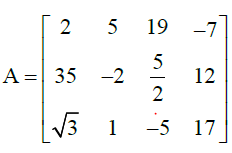write:
(i) The order of the matrix,
(ii) The number of elements,
(iii) Write the elements a13, a21, a33, a24, a23
Solution
(i) In the given matrix, the number of rows is 3 and the number of
columns is 4. Therefore, the order of the matrix is 3 × 4.
(ii) Since the order of the matrix is 3 × 4, there are 3 × 4 = 12 elements in it.
(iii) a13= 19, a21= 35, a33= −5, a24= 12, a23 =5/2

Question 2.
If a matrix has 24 elements, what are the possible orders it can have? What, if ithas 13 elements?
Solution
We know that if a matrix is of the order m × n, it has mn elements. Thus, to find all the possible orders of a matrix having 24 elements, we have to find all the ordered pairs of natural numbers whose product is 24.
The ordered pairs are: (1, 24), (24, 1), (2, 12), (12, 2), (3, 8), (8, 3),(4, 6), and (6, 4)
Hence, the possible orders of a matrix having 24 elements are:
1 × 24, 24 × 1, 2 × 12, 12 × 2, 3 × 8, 8 × 3, 4 × 6, and 6 × 4
If the matrix has 13 elements, then possible ordered pairs are (1, 13) and (13, 1) only.
Question 3.
If a matrix has 18 elements, what are the possible orders it can have? What, if it has 5 elements?
Solution
Like earlier question,
We must find all the ordered pairs of natural numbers whose product is 18.
The ordered pairs are: (1, 18), (18, 1), (2, 9), (9, 2), (3, 6,), and (6,3)
Hence, the possible orders of a matrix having 18 elements are:
1 × 18, 18 × 1, 2 × 9, 9 × 2, 3 × 6, and 6 × 3
(1, 5) and (5, 1) are the ordered pairs of natural numbers whose product is 5.
Hence, the possible orders of a matrix having 5 elements are 1 × 5 and 5 × 1.
Question 4.
Construct a 2 × 2 matrix, A = [aij], whose elements are given by:
i) aij =( i+j)2/2
ii) aij = i/j
iii) aij =( i+2j)2/2
Solution
The matrix will be given by the elements
a11, a12, a21, a22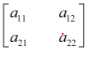i)  Substituting the values of i and j, we get the matrix as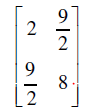ii) Substituting the values of i and j, we get the matrix as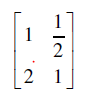iii) Substituting the values of i and j, we get the matrix as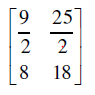Question 5.
Construct a 3 × 4 matrix, whose elements are given by:
(i)aij = (1/2) |-3i+j|
(ii) aij = 2i j
Solution
The matrix would be given by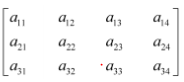So i in 1,2,3
J in 1,2,3,4
i)
a11 = (1/2) |-3(1)+1|=  1
a12= (1/2) |-3(1)+2|= ½
a13 = (1/2) |-3(1)+3|=0
a14 =(1/2) |-3(1)+4|=1/2
Similarly, can be calculated for other values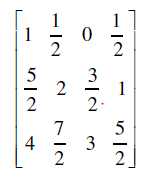ii)
a11 = 2(1) 1=1
a12 = 2(1) 2=0
a13 = 2(1) 3=-1
a14 = 2(1) 4=-2
Similarly, can be calculated for other values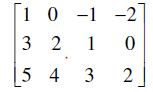Question 6.
Find the values of x, y and z from the following equations:
(i)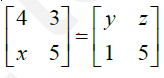(ii)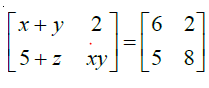(iii)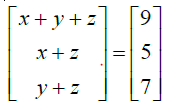Solution
As the given matrices are equal, their corresponding elements are also equal.
So comparing the values, we can easily the find the values of x,y,z
i) Comparing the corresponding elements, we get:
y=4
z=3
x=1
ii) Comparing the corresponding elements, we get:
x+y=6
or x=6-y  --(a)
5+z=5  or z=0
xy=8   --(b)
From (a) and  (b)
y(6-y) =8
or
y2 -6y+8 =0
or y =2 or 4
From (a)
x= 4 ,2
So the x,y,z values will be
( 2,4,0)  or (4,2,0)
iii)
Comparing the corresponding elements, we get:
x + y + z = 9 ... (a)
x + z = 5 ... (b)
y + z = 7 ... (c)
From (a) and (b), we have:
y + 5 = 9
or y = 4
Then, from (3), we have:
4 + z = 7
z = 3
Now
x + z = 5
x = 2
So values are
x = 2, y = 4, and z = 3
Question 7.
Find the value of a, b, c and d from the equation: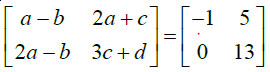Solution
As the given matrices are equal, their corresponding elements are also equal.
So comparing the values, we can easily the find the values of a,b,c  and d
a − b = −1 ... (1)
2a − b = 0 ... (2)
2a + c = 5 ... (3)
3c + d = 13 ... (4)
From (2), we have:
b = 2a
Then, from (1), we have:
a − 2a = −1
or a = 1
and  b = 2
Now, from (3), we have:
2 ×1 + c = 5
Or c = 3
From (4) we have:
3 ×3 + d = 13
9 + d = 13
Or d = 4
so a = 1, b = 2, c = 3, and d = 4
Question 8.
A = [aij]m × n is a square matrix, if
(A) m < n (B) m > n (C) m = n (D) None of these
Solution
It is known that a given matrix is said to be a square matrix if the number of rows is equal to the number of columns. Therefore, is a square matrix, if m = n.
Question 9.
Which of the given values of x and y make the following pair of matrices equal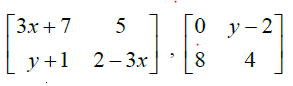a)  x= -1/3, y=7
b) Not possible to find
c) y=7, x=-2/3
d) x=1/3 ,y=2/3
Explanation
For the matrices to be equal, each of the element should be equal to corresponding elements
3x+7 =0 or x= -7/3
5=y-2 or y = 7
2-3x=4 or x =-2/3
We find that on comparing the corresponding elements of the two matrices, we get two different values of x, which is not possible.
Hence, it is not possible to find the values of x and y for which the given matrices are equal
Question 10.
The number of all possible matrices of order 3 × 3 with each entry 0 or 1 is:
(A) 27
(B) 18
(C) 81
(D) 512
Solution
The given matrix of the order 3 × 3 has 9 elements and each of these elements can be either 0 or 1.
Now, each of the 9 elements can be filled in two possible ways.
Therefore, by the multiplication principle, the required number of possible matrices is 29 = 512

Go back to Class 12 Main Page using below links